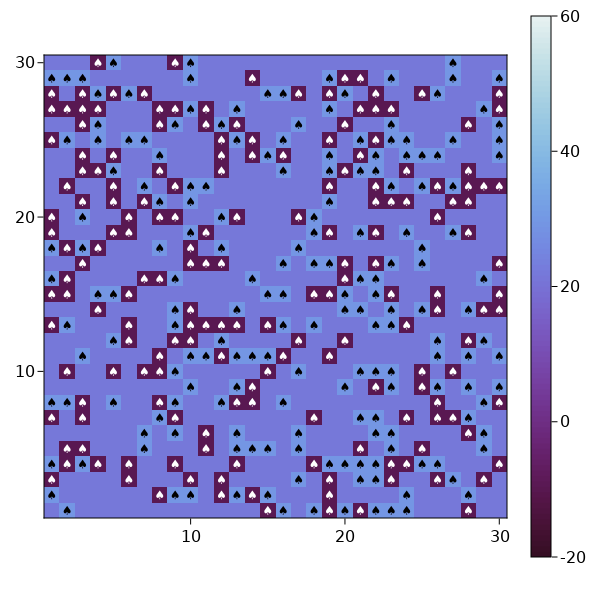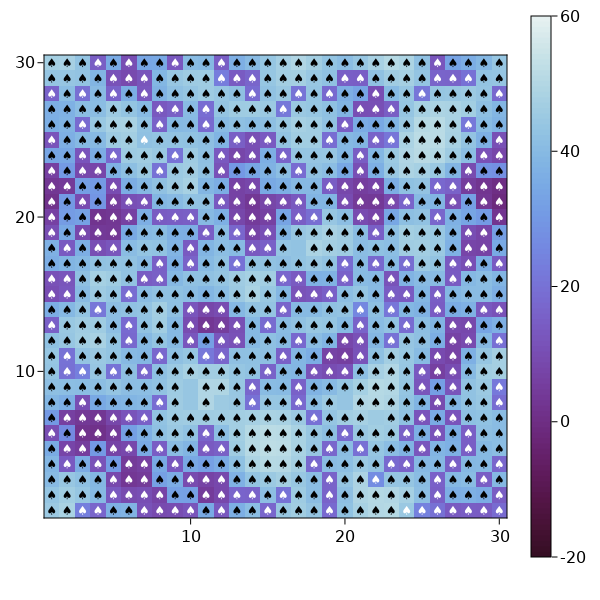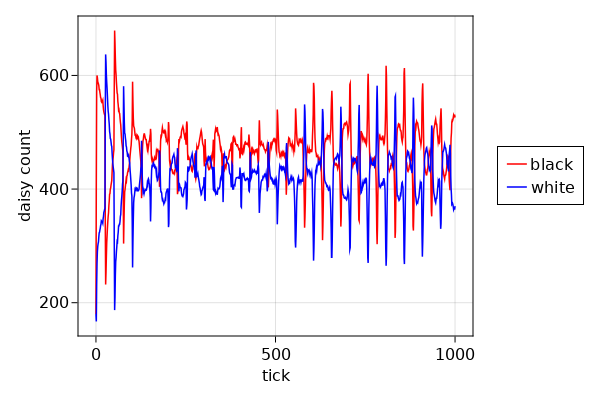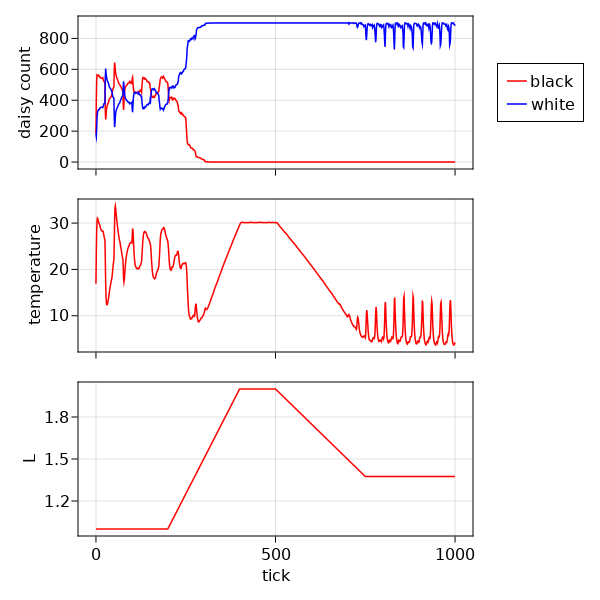# Daisyworld

Study this example to learn about

• Simple agent properties with complex model interactions
• Diffusion of a quantity in a GridSpace
• Including a "surface property" in the model
• counting time in the model and having time-dependent dynamics
• performing interactive scientific research

## Overview of Daisyworld

This model explores the Gaia hypothesis, which considers the Earth as a single, self-regulating system including both living and non-living parts.

Daisyworld is filled with black and white daisies. Their albedo's differ, with black daisies absorbing light and heat, warming the area around them; white daisies doing the opposite. Daisies can only reproduce within a certain temperature range, meaning too much (or too little) heat coming from the sun and/or surrounds will ultimately halt daisy propagation.

When the climate is too cold it is necessary for the black daisies to propagate in order to raise the temperature, and vice versa – when the climate is too warm, it is necessary for more white daisies to be produced in order to cool the temperature. The interplay of the living and non living aspects of this world manages to find an equilibrium over a wide range of parameter settings, although with enough external forcing, the daisies will not be able to regulate the temperature of the planet and eventually go extinct.

## Defining the agent types

Daisy has three values (other than the required id and pos for an agent that lives on a GridSpace. Each daisy has an age, confined later by a maximum age set by the user, a breed (either :black or :white) and an associated albedo value, again set by the user. Land represents the surface. We could make Land also have an albedo field, but in this world, the entire surface has the same albedo and thus we make it a model parameter.

Notice that the Land does not necessarily have to be an agent, and one could represent surface temperature via a matrix (parameter of the model). This is done in an older version, see file examples/daisyworld_matrix.jl. The old version has a slight performance advantage. However, the advantage of making the surface composed of agents is that visualization is simple and one can use the interactive application to also visualize surface temperature. It is also available from the Models module as Models.daisyworld.

using Agents
using Statistics: mean

mutable struct Daisy <: AbstractAgent
id::Int
pos::Dims{2}
breed::Symbol
age::Int
albedo::Float64 # 0-1 fraction
end

const DaisyWorld = ABM{<:GridSpace, Daisy};

## World heating

The surface temperature of the world is heated by its sun, but daisies growing upon it absorb or reflect the starlight – altering the local temperature.

function update_surface_temperature!(pos, model::DaisyWorld)
ids = ids_in_position(pos, model)
absorbed_luminosity = if isempty(ids) # no daisy
# Set luminosity via surface albedo
(1 - model.surface_albedo) * model.solar_luminosity
else
# Set luminosity via daisy albedo
(1 - model[ids].albedo) * model.solar_luminosity
end
# We expect local heating to be 80 ᵒC for an absorbed luminosity of 1,
# approximately 30 for 0.5 and approximately -273 for 0.01.
local_heating = absorbed_luminosity > 0 ? 72 * log(absorbed_luminosity) + 80 : 80
# Surface temperature is the average of the current temperature and local heating.
model.temperature[pos...] = (model.temperature[pos...] + local_heating) / 2
end
update_surface_temperature! (generic function with 1 method)

In addition, temperature diffuses over time

function diffuse_temperature!(pos, model::DaisyWorld)
ratio = get(model.properties, :ratio, 0.5) # diffusion ratio
npos = nearby_positions(pos, model)
model.temperature[pos...] =
(1 - ratio) * model.temperature[pos...] +
# Each neighbor is giving up 1/8 of the diffused
# amount to each of *its* neighbors
sum(model.temperature[p...] for p in npos) * 0.125 * ratio
end
diffuse_temperature! (generic function with 1 method)

## Daisy dynamics

The final piece of the puzzle is the life-cycle of each daisy. This method defines an optimal temperature for growth. If the temperature gets too hot or too cold, daisies will not wish to propagate. So long as the temperature is favorable, daisies compete for land and attempt to spawn a new plant of their breed in locations close to them.

function propagate!(pos, model::DaisyWorld)
ids = ids_in_position(pos, model)
if !isempty(ids)
daisy = model[ids]
temperature = model.temperature[pos...]
# Set optimum growth rate to 22.5 ᵒC, with bounds of [5, 40]
seed_threshold = (0.1457 * temperature - 0.0032 * temperature^2) - 0.6443
if rand(model.rng) < seed_threshold
# Collect all adjacent position that have no daisies
empty_neighbors = Tuple{Int,Int}[]
neighbors = nearby_positions(pos, model)
for n in neighbors
if isempty(ids_in_position(n, model))
push!(empty_neighbors, n)
end
end
if !isempty(empty_neighbors)
# Seed a new daisy in one of those position
seeding_place = rand(model.rng, empty_neighbors)
end
end
end
end
propagate! (generic function with 1 method)

And if the daisies cross an age threshold, they die out. Death is controlled by the agent_step function

function agent_step!(agent::Daisy, model::DaisyWorld)
agent.age += 1
agent.age >= model.max_age && kill_agent!(agent, model)
end
agent_step! (generic function with 1 method)

The model step function advances Daisyworld's dynamics:

function model_step!(model)
for p in positions(model)
update_surface_temperature!(p, model)
diffuse_temperature!(p, model)
propagate!(p, model)
end
model.tick += 1
solar_activity!(model)
end
model_step! (generic function with 1 method)

Notice that solar_activity! changes the incoming solar radiation over time, if the given "scenario" (a model parameter) is :ramp. The parameter tick of the model keeps track of time.

function solar_activity!(model::DaisyWorld)
if model.scenario == :ramp
if model.tick > 200 && model.tick <= 400
model.solar_luminosity += model.solar_change
end
if model.tick > 500 && model.tick <= 750
model.solar_luminosity -= model.solar_change / 2
end
elseif model.scenario == :change
model.solar_luminosity += model.solar_change
end
end
solar_activity! (generic function with 1 method)

## Initialising Daisyworld

Here, we construct a function to initialize a Daisyworld. We use fill_space! to fill the space with Land instances. Then, we need to know how many daisies of each type to seed the planet with and what their albedo's are. We also want a value for surface albedo, as well as solar intensity (and we also choose between constant or time-dependent intensity with scenario).

import StatsBase
import DrWatson: @dict
using Random

function daisyworld(;
griddims = (30, 30),
max_age = 25,
init_white = 0.2, # % cover of the world surface of white breed
init_black = 0.2, # % cover of the world surface of black breed
albedo_white = 0.75,
albedo_black = 0.25,
surface_albedo = 0.4,
solar_change = 0.005,
solar_luminosity = 1.0, # initial luminosity
scenario = :default,
seed = 165,
)

rng = MersenneTwister(seed)
space = GridSpace(griddims)
properties = @dict max_age surface_albedo solar_luminosity solar_change scenario
properties[:tick] = 0
properties[:temperature] = zeros(griddims)

model = ABM(Daisy, space; properties, rng)

# Populate with daisies: each position has only one daisy (black or white)
grid = collect(positions(model))
num_positions = prod(griddims)
white_positions =
StatsBase.sample(grid, Int(init_white * num_positions); replace = false)
for wp in white_positions
wd = Daisy(nextid(model), wp, :white, rand(model.rng, 0:max_age), albedo_white)
end
allowed = setdiff(grid, white_positions)
black_positions =
StatsBase.sample(allowed, Int(init_black * num_positions); replace = false)
for bp in black_positions
wd = Daisy(nextid(model), bp, :black, rand(model.rng, 0:max_age), albedo_black)
end

# Adjust temperature to initial daisy distribution
for p in positions(model)
update_surface_temperature!(p, model)
end

return model
end
daisyworld (generic function with 1 method)

## Visualizing & animating

Lets run the model with constant solar isolation and visualize the result

using InteractiveDynamics
using CairoMakie

model = daisyworld()
AgentBasedModel with 360 agents of type Daisy
space: GridSpace with size (30, 30), metric=chebyshev, periodic=true
scheduler: fastest
properties: temperature, solar_luminosity, max_age, surface_albedo, solar_change, tick, scenario

To visualize we need to define the necessary functions for abm_plot. We will also utilize its ability to plot an underlying heatmap, which will be the model surface temperature, while daisies will be plotted in black and white as per their breed. Notice that we will explicitly provide a colorrange to the heatmap keywords, otherwise the colormap will be continuously and automatically updated to match the underlying temperature values while we are animating the time evolution.

daisycolor(a::Daisy) = a.breed

plotkwargs = (
ac=daisycolor, as = 12, am = '♠',
heatarray = :temperature,
heatkwargs = (colorrange = (-20, 60),),
)
fig, _ = abm_plot(model; plotkwargs...)
figAnd after a couple of steps

Agents.step!(model, agent_step!, model_step!, 5)
fig, _ = abm_plot(model; heatarray = model.temperature, plotkwargs...)
figLet's do some animation now

model = daisyworld()
abm_video(
"daisyworld.mp4",
model,
agent_step!,
model_step!;
title = "Daisy World",
plotkwargs...,
)

Running this animation for longer hints that this world achieves quasi-equilibrium for some input parameters, where one breed does not totally dominate the other. Of course we can check this easily through data collection. Notice that here we have to define a function breed that returns the daisy's breed field. We cannot use just :breed to automatically find it, because in this mixed agent model, the Land doesn't have any breed.

black(a) = a.breed == :black
white(a) = a.breed == :white
adata = [(black, count), (white, count)]

model = daisyworld(; solar_luminosity = 1.0)

agent_df, model_df = run!(model, agent_step!, model_step!, 1000; adata)
figure = Figure(resolution = (600, 400))
ax = figure[1, 1] = Axis(figure, xlabel = "tick", ylabel = "daisy count")
blackl = lines!(ax, agent_df[!, :step], agent_df[!, :count_black], color = :red)
whitel = lines!(ax, agent_df[!, :step], agent_df[!, :count_white], color = :blue)
figure[1, 2] = Legend(figure, [blackl, whitel], ["black", "white"], textsize = 12)
figure## Time dependent dynamics

To use the time-dependent dynamics we simply use the keyword scenario = :ramp during model creation. However, we also want to see how the planet surface temperature changes and would be nice to plot solar luminosity as well. Thus, we define in addition

temperature(model) = mean(model.temperature)
mdata = [temperature, :solar_luminosity]
2-element Vector{Any}:
temperature (generic function with 1 method)
:solar_luminosity

And we run (and plot) everything

model = daisyworld(solar_luminosity = 1.0, scenario = :ramp)
agent_df, model_df =

figure = CairoMakie.Figure(resolution = (600, 600))
ax1 = figure[1, 1] = Axis(figure, ylabel = "daisy count", textsize = 12)
blackl = lines!(ax1, agent_df[!, :step], agent_df[!, :count_black], color = :red)
whitel = lines!(ax1, agent_df[!, :step], agent_df[!, :count_white], color = :blue)
figure[1, 2] = Legend(figure, [blackl, whitel], ["black", "white"], textsize = 12)

ax2 = figure[2, 1] = Axis(figure, ylabel = "temperature", textsize = 12)
ax3 = figure[3, 1] = Axis(figure, xlabel = "tick", ylabel = "L", textsize = 12)
lines!(ax2, model_df[!, :step], model_df[!, :temperature], color = :red)
lines!(ax3, model_df[!, :step], model_df[!, :solar_luminosity], color = :red)
for ax in (ax1, ax2); ax.xticklabelsvisible = false; end
figure## Interactive scientific research

Julia is an interactive language, and thus everything that you do with Agents.jl can be considered interactive. However, we can do even better by using our interactive application. In this example, rather than describing what solar forcing we want to investigate before hand, we use the interactive application, to control by ourselves, in real time, how much solar forcing is delivered to daisyworld.

So, let's make an InteractiveDynamics.abm_data_exploration.

using InteractiveDynamics, GLMakie, Random
model = daisyworld(; solar_luminosity = 1.0, solar_change = 0.0, scenario = :change)
AgentBasedModel with 360 agents of type Daisy
space: GridSpace with size (30, 30), metric=chebyshev, periodic=true
scheduler: fastest
properties: temperature, solar_luminosity, max_age, surface_albedo, solar_change, tick, scenario

The only significant addition to use the interactive application is that we make a parameter container for surface albedo and for the rate of change of solar luminosity, and add some labels for clarity.

params = Dict(
:surface_albedo => 0:0.01:1,
:solar_change => -0.1:0.01:0.1,
)
alabels = ["black", "white"]
mlabels = ["T", "L"]
2-element Vector{String}:
"T"
"L"

And we run it

fig, adf, mdf = abm_data_exploration(
model, agent_step!, model_step!, params;
)